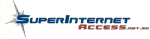tech stuff  |

# Tech Stuff - Electronics - Symbols and Equations

The following define the most widely used symbols and equations for capacitance (in series, parallel, quantity of stored electricity etc.), Ohm's Law (for DC circuits) and the decibel. We would also suggest you look at this 6 volume series of books by Tony R Kuphaldt 'Lessons in Electric Circuits' published under the OpenBook initiative (means its free).

### Symbols used in Electrical Equations

 Symbol Meaning C Capacitance measured in Farads (F): Degrees Celsius (temperature) D Dissipation factor; reciprocal of storage factor Q. d Thickness of dielectric material in a capacitor (measured in centimeters) dB Decibel; the ratio between two amounts of Power E Electromotive Force measured in Volts F Farads (measure of Capacitance); Degrees Farenheit (temperature) f Frequency measured in Hertz. G Conductance measured in siemens or mhos. I Current measured in amperes. J Energy, work or quantity of heat measured in Joules. L Inductance measured in henries. M Mutual inductance measured in henries. P Power measured in Watts. Q Quality or an inductor or Quantity of electricity stored (measured in coulombs). R Resistance measured in Ohms. Z Impedance measured in ohms.

## Electrical Equations

### Capacitance

In Parallel

Ctotal = C1 + C2 etc.

In Series

Ctotal = 1 / (1 / C1 + 1 / C2 etc.)

OR

1/ Ctotal = 1 / C1 + 1 / C2 etc.

Two Capacitors in series

Ctotal = C1 * C2 / C1 + C2

Capacitance of a Capacitor

C = 0.0885 KS (N - 1) / d

Quantity of Electricity Stored in a capacitor

Q = CE

### Ohm's Law for DC Circuits

The following equations are valid for DC circuits only. Where E = volts, P = watts (power), I = current, R = resistance.

1. I = E / R (current = volts / resistance)

2. I = [SR] P / R (current = square root of power / resistance)

3. I = P / E (current = power / volts)

4. R = E / I (resistance = volts / current)

5. R = P / I2 (resistance = power / current squared)

6. R = E2 / P (resistance = volts squared / power)

7. E = IR (volts = current * resistance)

8. E = P / I (volts = power / current)

9. E = [SR] PR (volts = square root power * resistance)

10. P = I2R (power = current squared * resistance)

11. P = EI (power = volts * current)

12. P = E2 / R (power = volts squared / resistance)

### Decibel

The Decibel - a relative measure of two values is calculated for electrical circuits as:Problems, comments, suggestions, corrections (including broken links) or something to add? Please take the time from a busy life to 'mail us' (at top of screen), the webmaster (below) or info-support at zytrax. You will have a warm inner glow for the rest of the day.

Tech Stuff

If you are happy it's OK - but your browser is giving a less than optimal experience on our site. You could, at no charge, upgrade to a W3C standards compliant browser such as Firefox

 Copyright © 1994 - 2023 ZyTrax, Inc. All rights reserved. Legal and Privacy site by zytraxweb-master at zytrax Page modified: January 20 2022.